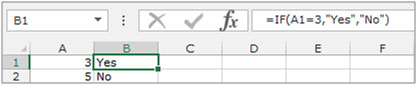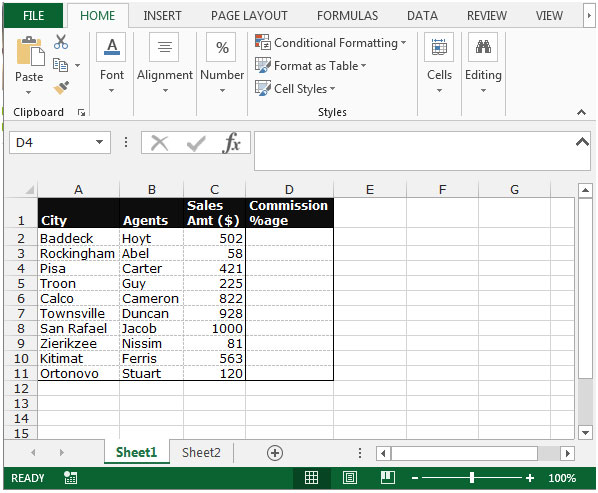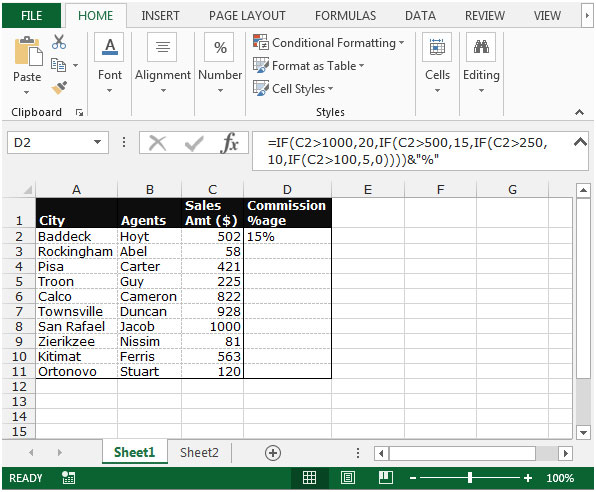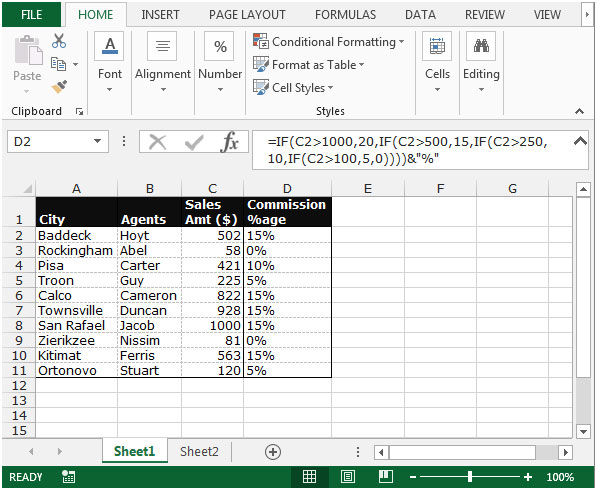# Calculate commission percentage based on a commission schedule in Microsoft Excel 2010

In this article we will learn how to calculate the commission percentage based on a commission schedule, we will use the IF function in Microsoft Excel 2010.

IF: - Checks whether a condition is met and returns one value if True and another value if False.

The syntax of “IF” function =if(logical test,[value_if_true],[value_if_false])

The function will perform a logical test and give the result based on whether its true or false.

For example, Cells A2 and A3 contain the numbers 3 and 5. If the number is 3, the formula should display “Yes”, else “No”.

=IF (A1=3,"Yes","No")Now let’s take an example to understand how to calculate the commission percentage based on a commission schedule.

We have sales data. Column A contains Agent’s city, Column B contains Agents, column C contains the sales amount. In column D, we need to calculatethe commission % age as per the criteria.The criteria is:-

1. If the sales amount is 1000,the commissionshouldbe 20%.
2. If the sales amount is 500,the commissionshould be 15%.
3. If the sales amount is 250,the commissionshould be 10%.
4. If the sales amount is 100,the commissionshould be 5%.
5. For sales less than 100,there should be no commission.

To calculatethe commission percentage based on the schedule, follow the below given steps:-

• Select the cell D2.

Write the formula
=IF(C2>1000,20,IF(C2>500,15,IF(C2>250,10,IF(C2>100,5,0))))&"%".

• Press Enter on your keyboard.
• The function will return the commission %age as per the criteria.• To return the commission %age for all the agents, copy the same formula by pressing the key Ctrl+C and paste in the range D3:D11 by pressing the key Ctrl+V on your keyboard.This is the way we can calculate the commission percentage based on a commission schedule in Microsoft Excel.

1.plz provied me formula my commission is .5% buT condition is min 2 and max 12

2.3.